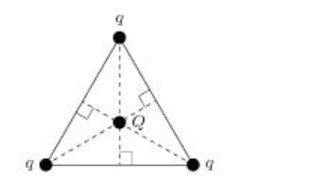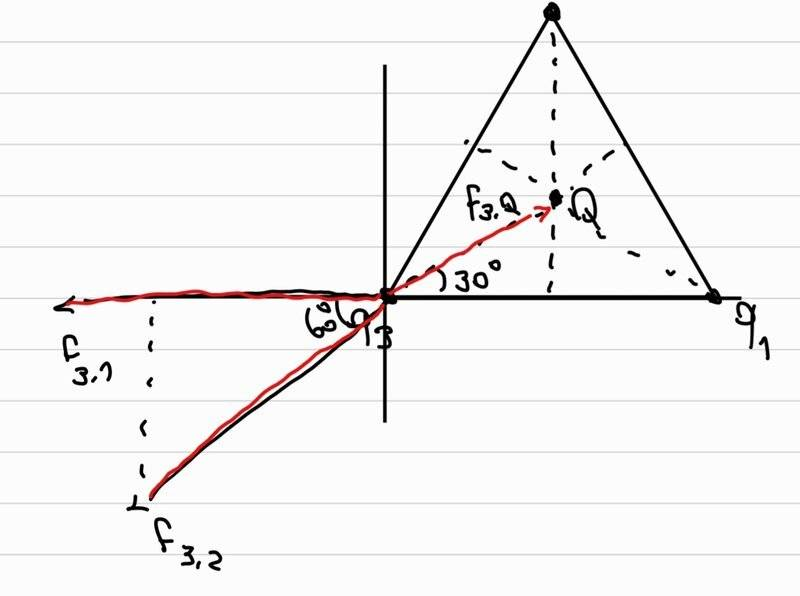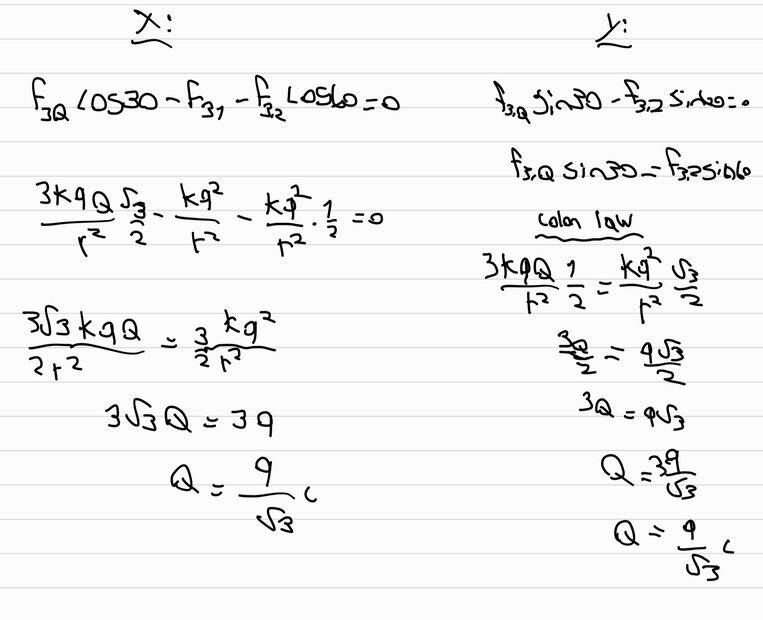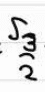# Coulomb's law — A negative charge balanced between 3 positive charges

yesmale4
Homework Statement:
Three equal positive charges, q = 8.5 μC each, are arranged at the vertices of equilateral triangle (see figure below). A negative charge, Q, is placed at the center of the triangle such that all four charges are in equilibrium.
Relevant Equations:
Ef=0
f=kq1q2/r^2
hello i would like to understand something, i found the right answer but there is still something i don't understand.
here is the figureand here is my correct solutionwhat i don't understand is why F(3,Q) is 3kQ/r^2
i mean why is the 3? i only calculat the force between q3 and Q so why the 3 before?

Last edited:

Homework Helper
Gold Member
2022 Award
You mean Coulomb's law. Not "colon law".

••malawi_glenn, PhDeezNutz and topsquark
yesmale4
You mean Coulomb's law. Not "colon law".
fixed thank you

Homework Helper
Gold Member
Homework Statement:: Three equal positive charges, q = 8.5 μC each, are arranged at the vertices of equilateral triangle (see figure below). A negative charge, Q, is placed at the center of the triangle such that all four charges are in equilibrium.
Relevant Equations:: Ef=0
f=kq1q2/r^2

hello i would like to understand something, i found the right answer but there is still something i don't understand.
here is the figure
View attachment 316259

and here is my correct solution

View attachment 316260
View attachment 316261

what i don't understand is why F(3,Q) is 3kQ/r^2
i mean why is the 3? i only calculat the force between q3 and Q so why the 3 before?
What does r represent in your solution? The distance from where to where?

•topsquark
Homework Helper
What is the question? (i can guess...)

I supposemeans ##\sqrt{3}\over 2## ?

You may want to learn some ##\LaTeX## (button at lower left of edit window). Or write and draw more unambiguously (the 60##^\circ## at the lower left looks more like 45##^\circ##, smack between 30##^\circ## and 60##^\circ##...)​

You seem to think that the sides of the triangle are ##r##. Fine, but then the distance ##qQ## is not ##r## !

##\ ##

•topsquark
Homework Helper
Gold Member
2022 Award
EDIT. Apologies @yesmale4. I misread your working. So this post is wrong and I've struck it through.

By the way @yesmale4, you have the incorrect unit in your answer. So your answer is wrong by a factor of one million!

Also, the value of q in the question is given to 2 significant figures. For consistency, your calculated value for Q should also have 2 significant figures.

Last edited:
Homework Helper
Gold Member
Also, the value of q in the question is given to 2 significant figures. For consistency, your calculated value for Q should also have 2 significant figures.
I don't see a calculated value. All I see is ##Q=\dfrac{q}{\sqrt{3}}## C. The issue is that there should be no units of Coulombs in the expression since it is a relation between magnitudes of charges.

Homework Helper
Gold Member
2022 Award
I don't see a calculated value. All I see is ##Q=\dfrac{q}{\sqrt{3}}## C. The issue is that there should be no units of Coulombs in the expression since it is a relation between magnitudes of charges.
Whoops. In haste I misread ##\frac q {\sqrt 3}## as ##\frac 9 {\sqrt 3}## (though that would have been an incorrect answer in any case).

•topsquark and kuruman
Staff Emeritus
Homework Helper
Gold Member
Homework Statement:: Three equal positive charges, q = 8.5 μC each, are arranged at the vertices of equilateral triangle (see figure below). A negative charge, Q, is placed at the center of the triangle such that all four charges are in equilibrium.
Relevant Equations:: Ef=0
f=kq1q2/r^2

hello i would like to understand something, i found the right answer but there is still something i don't understand.
here is the figure
View attachment 316259

and here is my correct solution

[ ATTACH type="full" alt="aa.jpg"]316260[/ATTACH]
[ ATTACH type="full" alt="aaa.jpg"]316261[/ATTACH]

what I don't understand is why F(3,Q) is 3kQ/r^2
i mean why is the 3? i only calculated the force between q3 and Q so why the 3 before?
This seems rather strange. It's your solution, but you do not know where the 3 comes from.

Notice that the distance from q1 to q2 , etc. is not the same as the distance from each q to the central Q.

If you let R be the distance from each q to the charge Q in the center you should find that, ##\dfrac{r/2}{R} =\cos(30^\circ)## .

Last edited by a moderator:
•